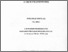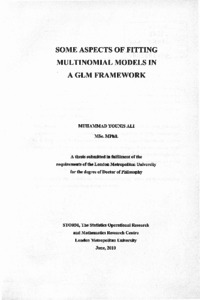# Some aspects of fitting multinomial models in a GLM framework

Ali, Muhammad Younis (2010) Some aspects of fitting multinomial models in a GLM framework. Doctoral thesis, London Metropolitan University.Preview
Text
540613.pdf - Published Version

## Abstract / Description

This thesis discusses how an iteratively re - weighted least squares algorithm can be used to fit a multinomial regression model, with logit link function or own link functions, with any number of explanatory variables. The responses of each individual can be aggregated and the data can then be represented in a contingency table as are given in examples used in this study.

A satisfying aspect of the iteratively re-weighted least squares (IRLS) algorithm gives for fitting a multinomial regression model is that the calculations only require a program which can handle ordinary least squares and hence can be handled by a range of standard statistical software.

The approach in this thesis applies an interesting and simple form of the Cholesky decomposition to a matrix that consists of diagonal sub - matrices to find the iterative weight matrix W ij. This method requires no matrix algebra facilities as all the calculations are carried out in an array format. This makes it amenable to implementation in most statistical software, including GLIM and shows how to fit a multinomial logit model without recourse to the Poisson trick approach of Francis et al. (1992).
The method given here also allows us to find the ‘hat - matrix’ as is needed in the calculation of leverages and Cook’s distances. These statistics in general can be used for diagnostic purposes or to detect the influential observations but in multinomial models the ‘hat - matrix’ may have a very little or no use at all for detecting any inappropriate observation. Our approach is in contrast to any potential naive use of the Poisson trick approach of Francis et al. (1992) model that would then produce inappropriate leverages and Cook’s distances.

We check that our approach gives exactly the same scaled deviance with correct degrees of freedom, parameter estimates and standard errors as are obtained from the Poisson trick approach with some minor rounding errors. Our approach has the freedom to consider any number of response levels or explanatory variables for fitting a multinomial regression model.

The method given here in this research for the multinomial data is quite general. It allows us to use different link functions, as is explained in more detail in chapter 4. We concentrate on use of so - called Box - Cox links. Interval estimates for different parameters in these user - defined ‘own’ link functions are also given in section 4.6, although, in the first three chapters the main concentration is on the logit link functions.

Chapter 5 gives a suggestion as to how we can get sharper convergence by using re - parameterisation of the design matrix. This is useful as in some cases as without such a modified design matrix we may not get convergence even after 1000 iterations. Chapter 6 indicates with examples that the theory developed in the previous chapters works well in a more general form of a multinomial data and discusses how the startup values must be considered with some knowledge of the data. Chapter 7 suggests how to calculate the likelihood influence measure of Cook (1986) by the idea of a single case i deletion for our fitting of multinomial models and for the Poisson trick approach of Francis et al. (1992). This can be further used to detect any influential observations for the regression parameter estimate of β only. Chapter 8 presents the conclusions and gives some guidelines for further extensions of the ideas presented in this thesis.

Item Type: Thesis (Doctoral) uk.bl.ethos.540613 least squares; algorithms; multinomial regression model; multinomial models; regression analysis 500 Natural Sciences and Mathematics > 510 Mathematics School of Computing and Digital Media Chiara Repetto 15 Feb 2023 14:25 01 Mar 2023 16:42 https://repository.londonmet.ac.uk/id/eprint/8302View Item